# Line following robot with OpenCV and contour-based approach

## Light problem

`def balance_pic(image):    global T    ret = None    direction = 0    for i in range(0, tconf.th_iterations):        rc, gray = cv.threshold(image, T, 255, 0)        crop = Roi.crop_roi(gray)        nwh = cv.countNonZero(crop)        perc = int(100 * nwh / Roi.get_area())        if perc > tconf.white_max:            if T > tconf.threshold_max:                break            if direction == -1:                ret = crop                break            T += 10            direction = 1        elif perc < tconf.white_min:            if T < tconf.threshold_min:                break            if  direction == 1:                ret = crop                break            T -= 10            direction = -1        else:            ret = crop            break      return ret`

## Make driving decisions

`def check_shift_turn(angle, shift):    turn_state = 0    if angle < tconf.turn_angle or angle > 180 - tconf.turn_angle:        turn_state = np.sign(90 - angle)    shift_state = 0    if abs(shift) > tconf.shift_max:        shift_state = np.sign(shift)    return turn_state, shift_statedef get_turn(turn_state, shift_state):    turn_dir = 0    turn_val = 0    if shift_state != 0:        turn_dir = shift_state        turn_val = tconf.shift_step if shift_state != turn_state else tconf.turn_step    elif turn_state != 0:        turn_dir = turn_state        turn_val = tconf.turn_step    return turn_dir, turn_val`
`while(True):            a, shift = get_vector()            if a is None:              # there is some code omitted related to line finding              break            turn_state, shift_state = check_shift_turn(a, shift)            turn_dir, turn_val = get_turn(turn_state, shift_state)            if turn_dir != 0:                turn(turn_dir, turn_val)            else:                time.sleep(tconf.straight_run)`

## Algorithm settings

`## Picture settings# initial grayscale thresholdthreshold = 120# max grayscale thresholdthreshold_max = 180#min grayscale thresholdthreshold_min = 40# iterations to find balanced thresholdth_iterations = 10# min % of white in roiwhite_min=3# max % of white in roiwhite_max=12## Driving settings# line angle to make a turnturn_angle = 45# line shift to make an adjustmentshift_max = 20# turning time of shift adjustmentshift_step = 0.125# turning time of turnturn_step = 0.25# time of straight runstraight_run = 0.5# attempts to find the line if lostfind_turn_attempts = 5# turn step to find the line if lostfind_turn_step = 0.2# max N of iterations of the whole trackingmax_steps = 100`

--

--

--

## More from Constantin Toporov

Working on artificial intelligence and computer vision applied to sports.

Love podcasts or audiobooks? Learn on the go with our new app.

## Ensemble learning — stacking models with scikit-learn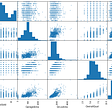## Natural Language Processing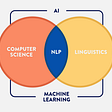## Synthetic data to develop a trustworthy autonomous driving system | Chapter 4## ML for Data Platform Operations## My First Work With PyTorch## A Low Cost Vehicle Localization System based on HD Map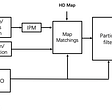## Hyperparameter tuning using GridSearchCV and RandomizedSearchCV## Understanding neural networks 2: The math of neural networks in 3 equations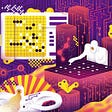## Constantin Toporov

Working on artificial intelligence and computer vision applied to sports.

## IMAGE PROCESSING -COMBINING TWO IMAGES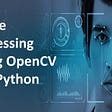## Air Mouse: Doing Mouse Operations Using Finger Gestures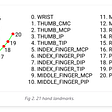## Multi-person pose estimation with Mediapipe and Yolov5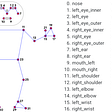## How to Install OpenCV with GPU support?